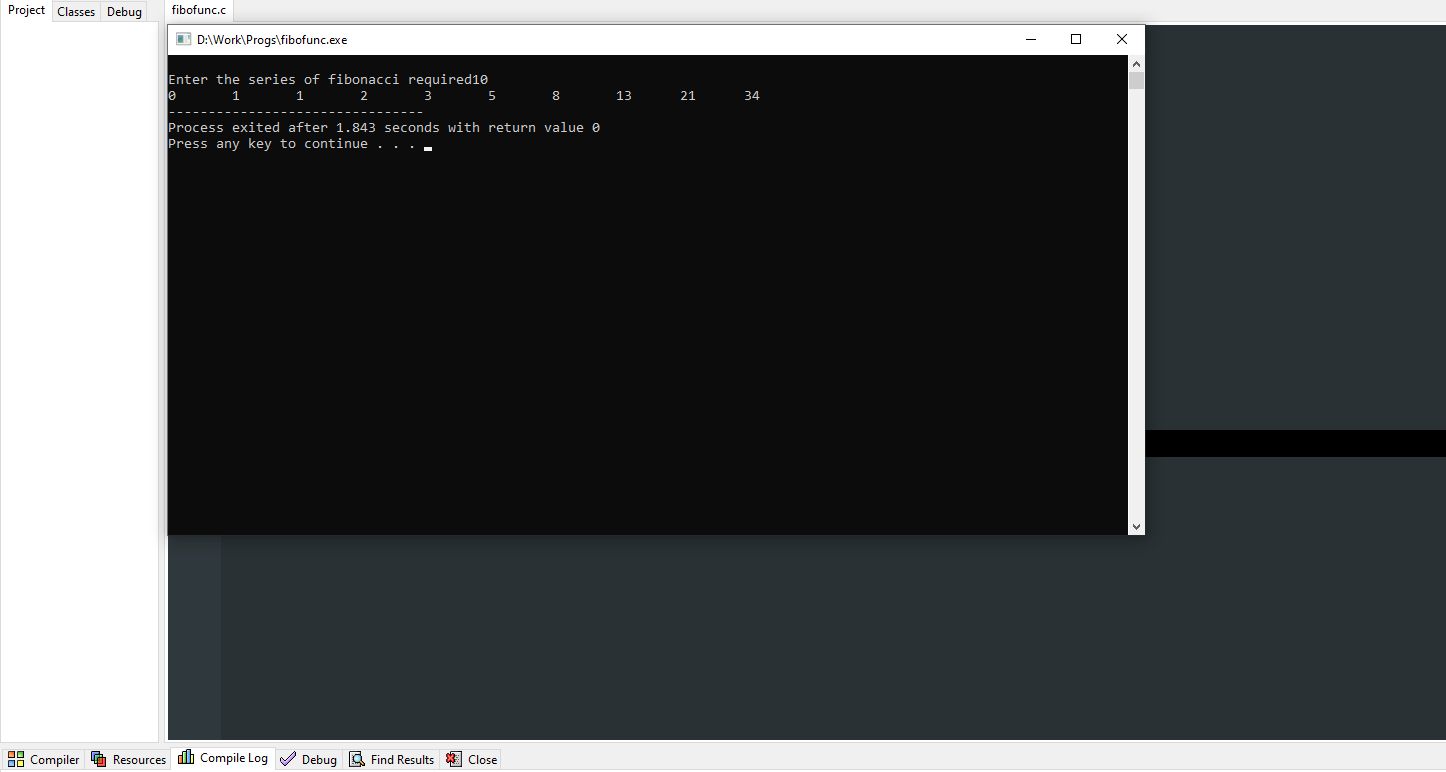# How does Fibonacci recursion work?

Here I am going to discuss about Fibonacci Series in Recursion form.

Means,

How Fibonacci Recursion Work?

First gone through,

## What is recursion?

A recursion is a function which calls itself and keeps on repeating until the condition is satisfied for the termination of the process.

### Syntax:

```void recur()
{
recur();
}
int main()
{
recur();
}```

## What is a Fibonacci series?

A Fibonacci is a series of number which is represented as:

0,1,1,2,3,5,8,13,21,34…..

It is generated by adding the present number with its number at the previous place.

```0,1 : 0+1=1
0,1,1 : 1+1=2
0,1,1,2 : 2+1=3
0,1,1,2,3 : 3+2=5
0,1,1,2,3,5 : 5+3=8
and so on```

This is how a Fibonacci series is generated. We will see how this series can be generated and its working using recursion in this article.

### Explanation:

1. We will take an integer i for a loop where the loop will work from 0 to n i.e., to the number of series desired by the user and the loop gets incremented by 1.
2. Inside the loop it will be printing the value on calling the function fibo which will return the series.
3. In the function fibo if the value of i which is called with the function tends to be 0 then it will return the value 0 which gets printed and then if the value tends to be 1 then it will return the value 1.
4. Moreover in the function a recursion process is called as
``fibo(i-1)+fibo(i-2)``

which states that the resultant value of i-1 and i-2 is added where the function for i-1 runs separately and the function for i-2 runs separately and in the end the result of both the functions are added and returned.

1. Printf function prints the value of the content returned from the fibo function.
2. When the value of i reaches equal to or greater than n then the loop gets terminated.

Implementation of above steps:

```#include<stdio.h>
int fibo(int i)
{
if(i==0)
return 0;
if(i==1)
return 1;
return (fibo(i-1)+fibo(i-2));
}
int main()
{
int i,n;
printf("\nEnter the series of fibonacci required");
scanf("%d",&n);
for (i=0;i<n;i++)
printf("%d\t",fibo(i));
return 0;
}
```

Output:The above program and explanation show how a Fibonacci series works using recursion. There may be different logics among which the above shown is a type.##### Soham Malakar

Hello my name is Soham Malakar am currently pursuing BCA

Coding is one of my favorite hobbies.
I love to code and an enthusiast to learn different types of programming languages.
I love to get intoxicated in the world of technology and video games.
A quick learner possessing better results.

Articles: 40